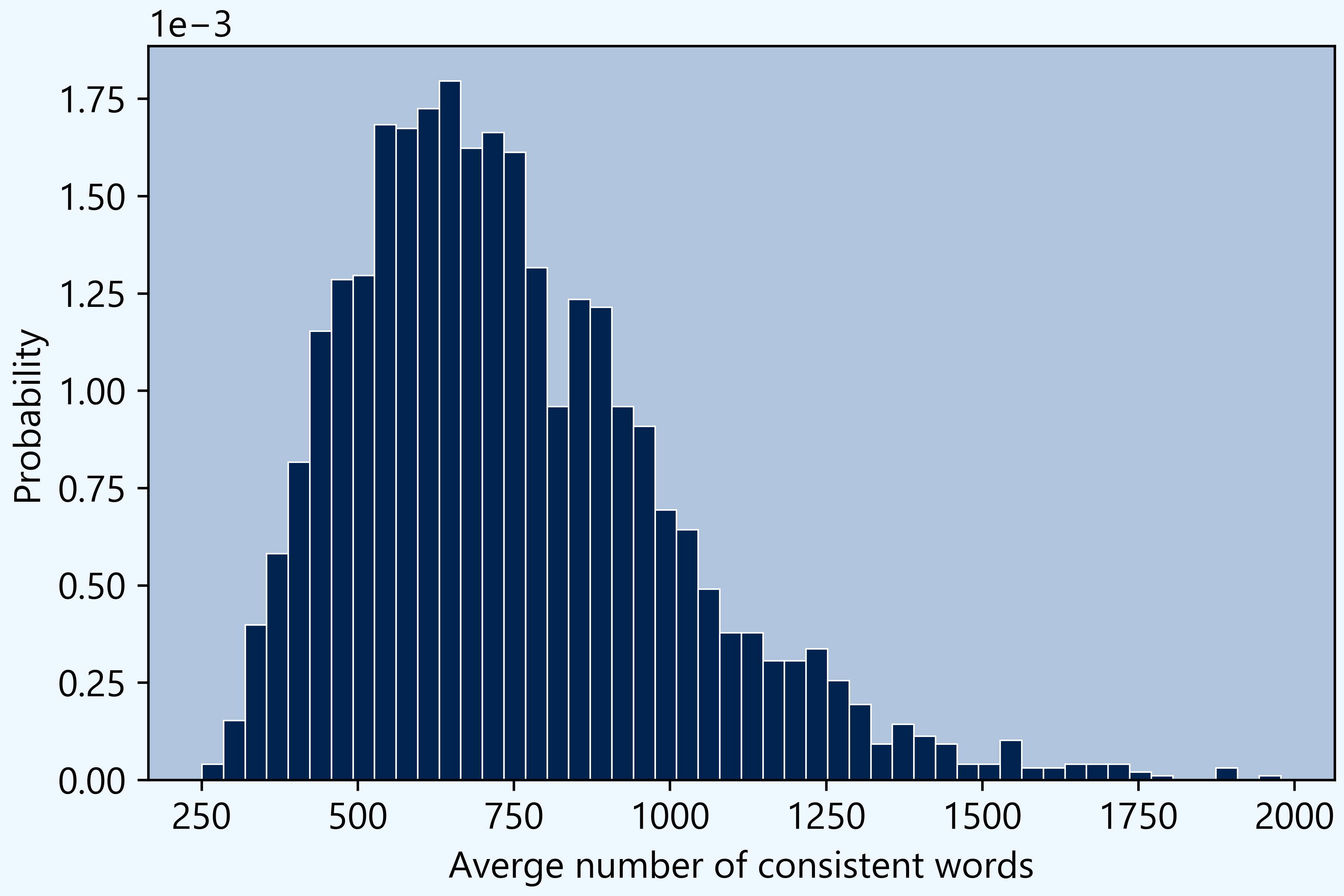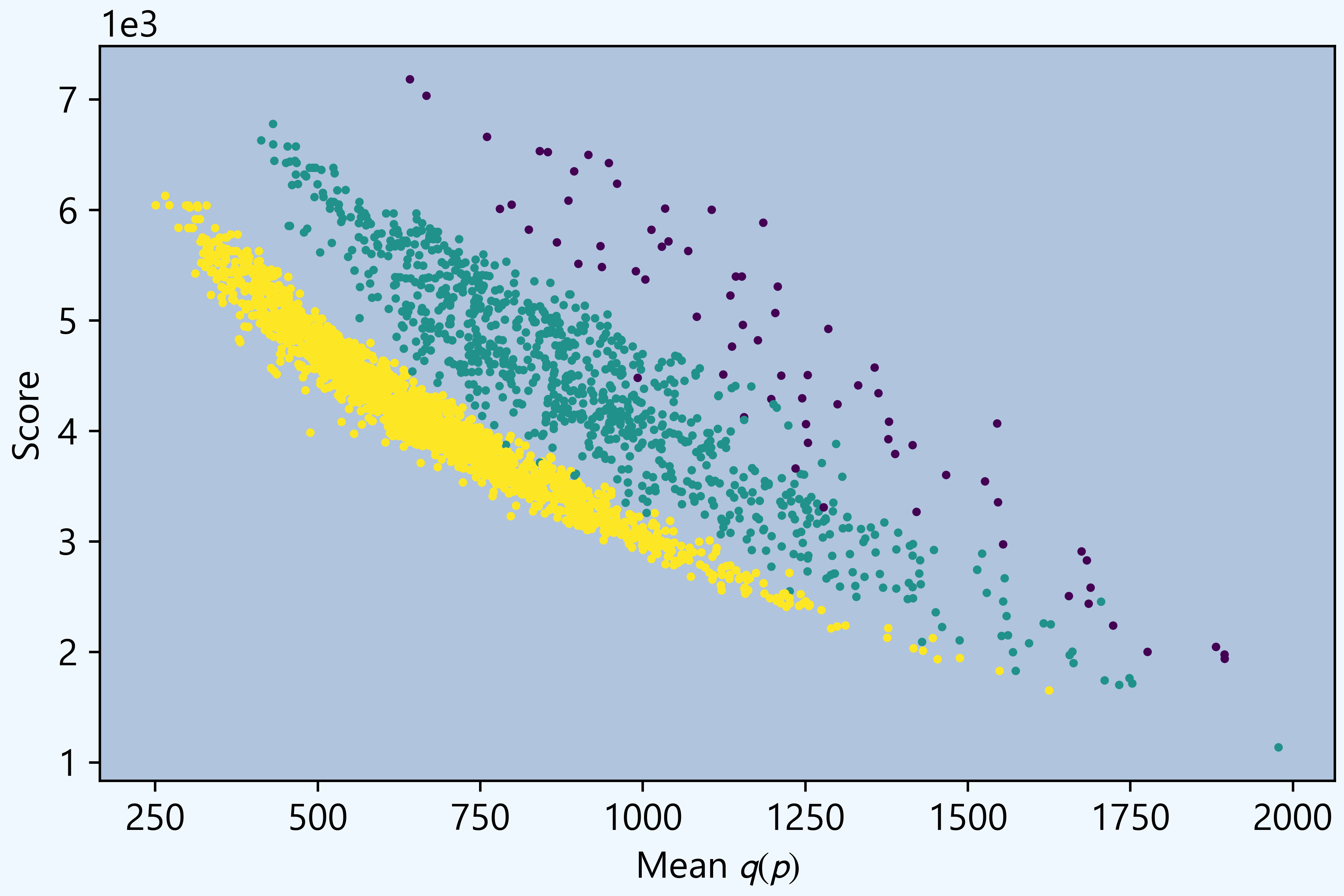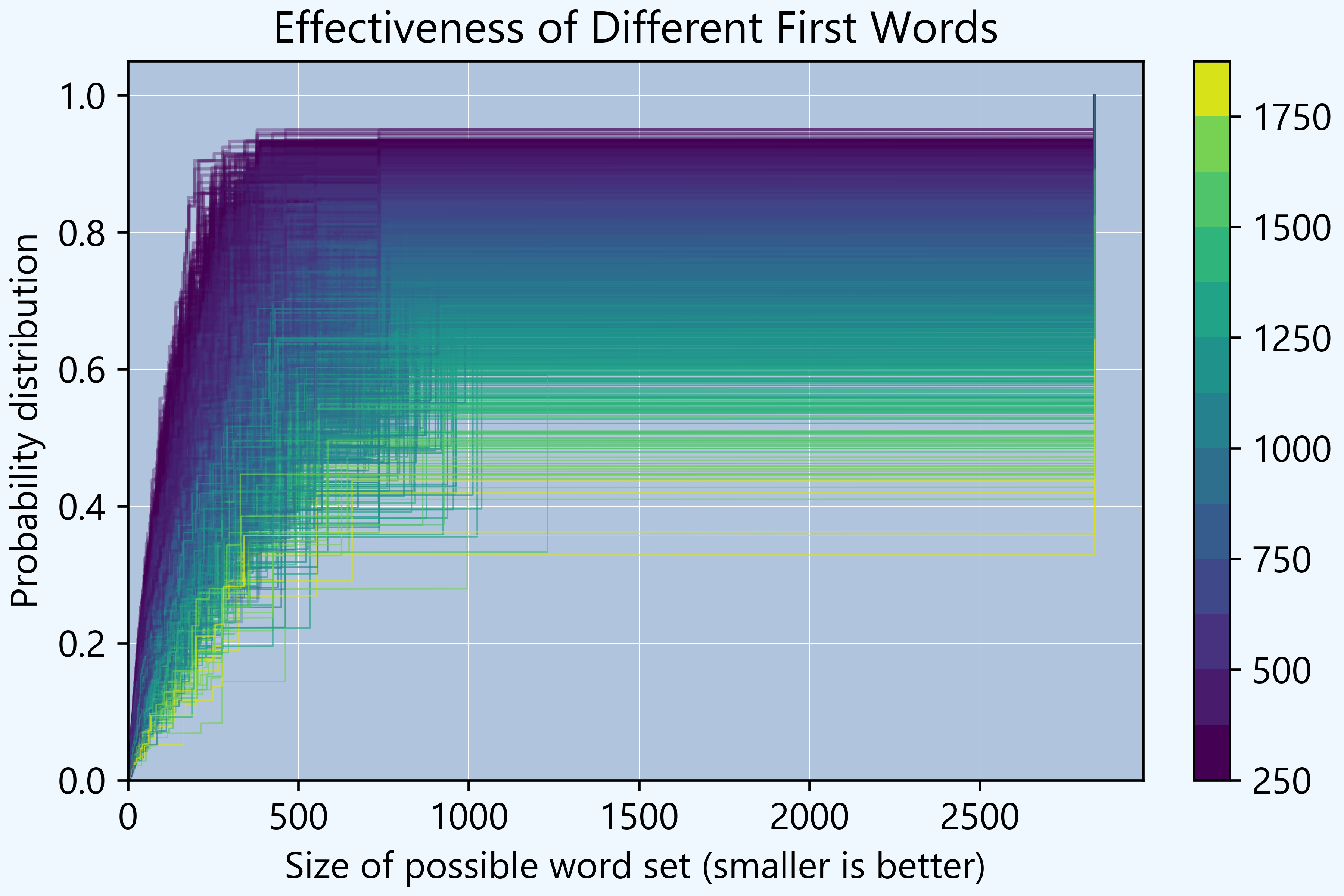# Wordle: the Best Start

Wordle is a popular word game. You have six chances to guess a five-letter word. For each guess, you learn how many letters are in the right place, and how many are in the word but in a different place. For example, if the unknown word is

• sound

and you guess

• doubt

then you learn that the unknown word

• has second letter o,
• contains d, but it is not the first letter, and
• does not contain u, b, or t.

Playing Wordle, you immediately wonder what is the most informative first word to guess?

To model a solution, assume that the unknown word $$W$$ is selected from a set of possible words $$\mathcal{W}$$. If you guess $$g\in \mathcal{W}$$, then you learn that $$W$$ is in a subset $$\mathcal{W}(g, W)\subset \mathcal{W}$$ of consistent words. An informative guess is one where $$\mathcal{W}(g, W)$$ is small for any unknown $$W$$. You could guess $$g=W$$, in which case there is just one consistent word. But generally, you will not be so lucky, and there will still be many possible answers after your first guess.

Guessing fuzzy, which contains a rare letter and uses only four distinct letters, generally (for most $$W$$) won’t narrow down the set of possible answers by much. It is a uninformative starting guess. On the other hand, guessing their reveals more information: it contains five common letters, and the set of consistent words will usually be smaller.

The average size of $$\mathcal{W}(g, W)$$ over all unknown $$W$$ is a measure of the information in the guess $$g$$. In symbols, define the quality of the guess $$g$$ as $q(g)=\mathsf E_W[|\mathcal{W}(g,W)|]$ where $$\mathsf E_W$$ denotes the average across all unknown $$W$$, and $$|\mathcal{W}(g,W)|$$ is the number of consistent words. The most informative possible word is the one with the lowest $$q$$.

Computing $$q(g)$$ involves three steps. Given the guess $$g$$:

1. For each possible actual word $$W$$, determine which letters of $$g$$ are in the right place, which are included but in the wrong place, and which are excluded.
2. Count how many words $$W'\subset\mathcal W$$ are consistent with the information in 1, giving $$|\mathcal{W}(g,W)|$$.
3. Average step 2 across all unknown $$W$$ to compute $$q(g)$$

If $$\mathcal{W}$$ contains $$n$$ words, each of these steps involves an order of $$n$$ operations, giving $$n^3$$ in all. Computing $$g$$ is very computationally intensive.

Here are the ten most informative first Wordle words based on a collection of 2,837 five-letter words (word list Match 12 from mynl.com).

Guess $$g$$ Mean $$q(g)$$
arise 251
raise 251
arose 266
aries 272
aisle 286
stare 297
tesla 301
aster 302
least 302
laser 303

These words are substantially better than an average guess, which has a mean nearly three times higher: 746. Mean has a standard deviation of 260. The ten worst words are in the next table.

Guess $$g$$ Mean $$q(g)$$
huffy 1711
bobby 1724
jazzy 1733
buggy 1749
jiffy 1753
fluff 1777
puppy 1882
mummy 1895
yummy 1895
fuzzy 1978

The less informative starting words contain rare letters and doubles.

Here is the distribution of means across all words.Histogram of the average number of consistent words, across all first word guesses.

The histogram shows that words like arise are significantly better than a random guess. They are in the extreme left tail of the distribution.

## A shortcut

Computing the exact most informative Wordle word is very time-consuming. Is there a shortcut? Words made out of letters that occur more frequently in $$\mathcal W$$ should contain more information, suggesting scoring words by letter frequency. Implementing this approach and comparing the resulting score to the exact mean gives the following plot. The three-color groups correspond to the number of distinct letters: yellow dots correspond to words with five distinct letters, green to four, and purple to three.

The graph shows that score is a reasonable proxy for the exact $$q$$.Comparison of mean and score measures of word guess information. Yellow dots are guesses with five distinct letters, green four, and blue three.

Words with four distinct letters score higher because they can double up the most common letters. Overall, eases has the highest word-frequency score, 7,181, but its mean is 642.

Here are the top ten outcomes sorted by score, using five letter words.

Guess $$g$$ Mean $$q(g)$$ Letters Letter Score
arose 266 5 6,127
raise 251 5 6,041
arise 251 5 6,041
aries 272 5 6,041
stare 297 5 6,039
rates 329 5 6,039
tears 315 5 6,039
aster 302 5 6,039
earls 316 5 6,020
laser 303 5 6,020

The top-ten score list includes all top-ten mean words except least, (it substitutes earls). In practice, using the frequency-based score seems a reasonable substitute for the exact mean. Using scores remains practical for larger $$\mathcal{W}$$ than the exact method.

## DistributionsDistribution of the number of possible words across all first guesses.

The graph above shows the distribution of the size of the possible set after one guess, across all guesses. After an optimal or near optimal first guess, there is an 80% chance that you rule out about 90% of words. The measure $$q$$ is based on the mean, but other choices, such as a percentile could also be used.

## Bayes

We have used Bayes’s theorem to finding the most informative first word. The prior distribution is uniform over $$\mathcal W$$. Conditional on the information in the first guess, the posterior distribution is uniform over the smaller set $$\mathcal W(g, W)$$. The model we have described could be extended to allow for the case where the unknown words are not selected randomly. An interesting question is to determine a rule for picking W that makes the puzzle the hardest to solve.

## Most difficult word to guess

Once the sizes of the sets of possible words $$|\mathcal W(g, W)|$$ have been computed for all $$g$$ and $$W$$ we can turn the information around and ask for the most difficult word $$W$$ to guess. Guessing difficulty is measured by the average $$|\mathcal W(g, W)|$$ over guesses $$g$$, with larger values corresponding to words that are harder to guess. The list of hard words to guess is similar to the list of uninformative first guess words. Here are the top ten.

Guess $$g$$ Mean $$\mathsf E_g|\mathcal W(g, W)|$$
huffy 1,681
bobby 1,682
jiffy 1,692
woozy 1,692
fluff 1,715
buggy 1,731
puppy 1,864
yummy 1,876
mummy 1,878
fuzzy 1,958

posted 2022-02-01 | tags: words, regular expressions